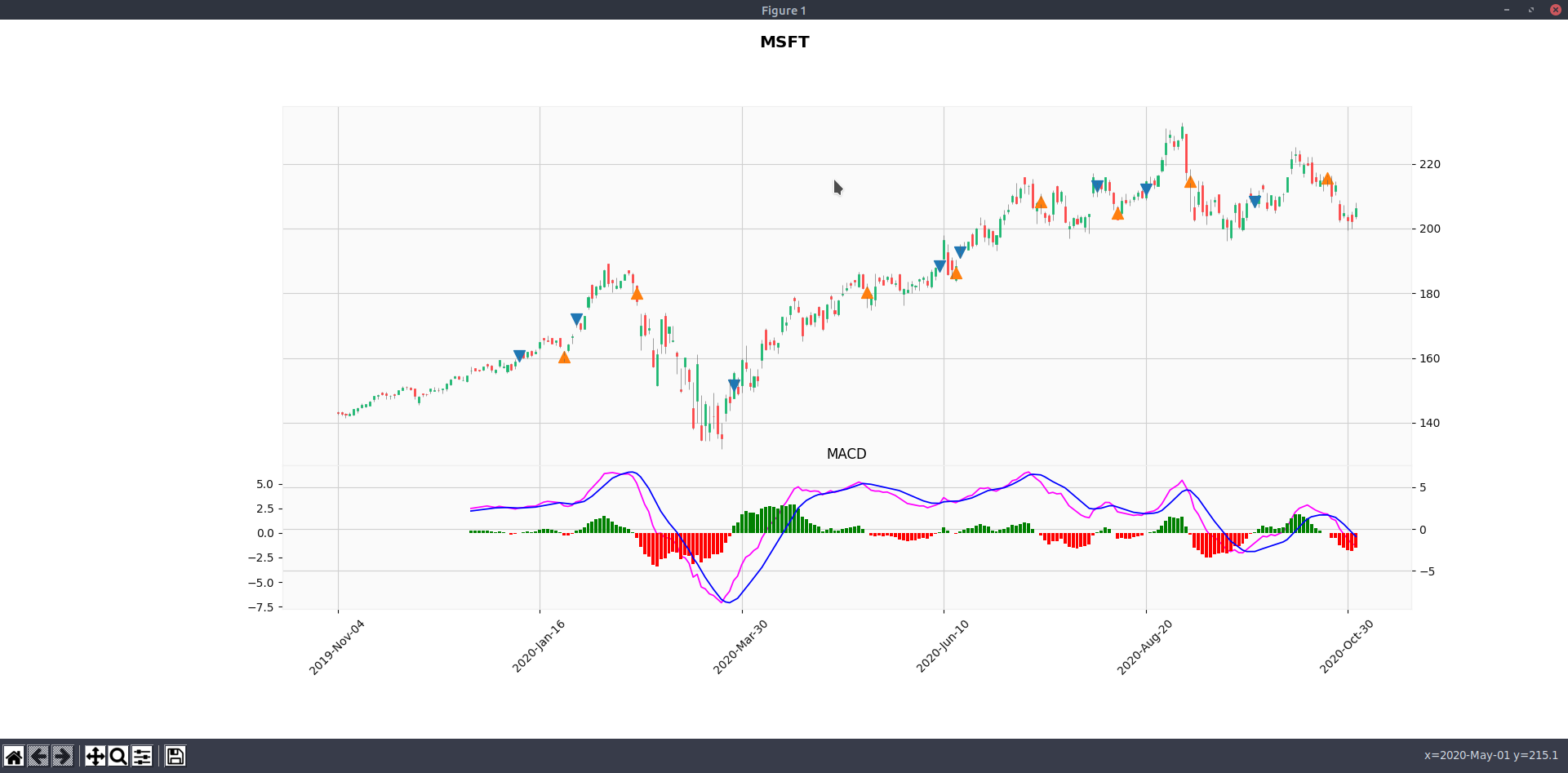# Test Trading Performance Based on MACD (Python)

November 3, 2020

## Note

• Use MACD signal only: Buy when Signal line cross above MACD line (Postive Histogram), Sell when Signal line cross below MACD line (Negative Histogram)
• Plot candle chart and MACD chart for better visualization
• Plot buy and sell points on the chart
• Print buy/sell transactions and profit/loss
• Meassure performance

## Code

Install libraries

``````pip install yfinance
pip install mplfinance
``````
``````import yfinance as yf
import mplfinance as mpf
import talib as ta
import numpy as np
import pandas as pd
import math

ticker_name = 'MSFT'
yticker = yf.Ticker(ticker_name)
data = yticker.history(period="1y") # max, 1y, 3mo

# macd
data["macd"], data["macd_signal"], data["macd_hist"] = ta.MACD(data['Close'])

# plot macd
macd_plot = mpf.make_addplot(data["macd"], panel=1, color='fuchsia', title="MACD")
colors = ['g' if v >= 0 else 'r' for v in data["macd_hist"]]
macd_hist_plot = mpf.make_addplot(data["macd_hist"], type='bar', panel=1, color=colors) # color='dimgray'

sell_plot = mpf.make_addplot(sell_signals, type='scatter', marker='^', markersize=100, panel=0)

# print buy/sell transaction and stats
print_performance_summary(signals)

# plot candle chart and all
plots = [macd_plot, macd_signal_plot, macd_hist_plot, buy_plot, sell_plot]
mpf.plot(data, type='candle', style='yahoo', addplot=plots, title=f"\n{ticker_name}", ylabel='')
``````
``````def detect_macd_signals(data):
"""Use MACD cross-over to decide buy/sell

Args:
data: panda DataFrame with OHLC with MACD data

Return:
signals: buy/sell transaction for summary printing
"""

sell_signals = [np.nan]
signals = []
last_signal = None

for i in range(1, len(data)):
if data['macd_hist'][i-1] < 0 and data['macd_hist'][i] > 0:
price = (data['Open'][i] + data['Close'][i]) / 2
signals.append({
'date': data.index[i],
'price': price
})
sell_signals.append(np.nan)
elif data['macd_hist'][i-1] > 0 and data['macd_hist'][i] < 0 and last_signal == 'buy':
price = (data['Open'][i] + data['Close'][i]) / 2
sell_signals.append(price)
last_signal = 'sell'
signals.append({
'date': data.index[i],
'action': 'sell',
'price': price
})
else:
sell_signals.append(np.nan)

``````
``````def print_performance_summary(signals):

Args:
"""
pairs = zip(*[iter(signals)]*2)
rows = []

profit_count = 0
profit_pct_avg = 0

if profit > 0:
profit_count += 1
profit_pct_avg += profit_pct

row = {
'profit': profit,
'profit_pct': "{0:.2%}".format(profit_pct)
}
rows.append(row)

df =  pd.DataFrame(rows, columns=['buy_date', 'duration', 'profit', 'profit_pct'])
with pd.option_context('display.max_rows', None, 'display.max_columns', None):
print(df)

total_transaction = math.floor(len(signals) / 2)
stats = {
'total_transaction': total_transaction,
'profit_rate': "{0:.2%}".format(profit_count / total_transaction),
'avg_profit_per_transaction': "{0:.2%}".format(profit_pct_avg / total_transaction)
}
for key, value in stats.items():
print('{0:30}  {1}'.format(key, value))
``````

## Result

Output of `print_performance_summary` for `MSFT`

• We manage to do 8 pairs of trade (buy/sell) in 1 year
• 5/8 (62.50%) trades are profitable
• Average profit per trade is 3.89%, with most profitable trade making 18.98%, while the worst lost -3.96%.
``````    buy_date  duration     profit profit_pct
0 2020-01-09        18  -0.248023     -0.15%
1 2020-01-30        22   7.896236      4.59%
2 2020-03-26        48  28.745260     18.98%
3 2020-06-09         6  -2.134837     -1.13%
4 2020-06-16        29  15.532458      8.06%
5 2020-08-04         7  -8.439593     -3.96%
6 2020-08-20        15   2.615005      1.23%
7 2020-09-29        24   7.324997      3.52%

total_transaction               8
profit_rate                     62.50%
avg_profit_per_transaction      3.89%
``````## What’s Next

• We assume the cross-over of MACD and Signal line is the buy/sell trigger. We could run more tests to determine whether delaying N day after cross-over would yield better result.
• Maybe we want to consider the weightage if the signal line is above 0 or below 0.
• We should test with more stocks, either S&P 500 or NASDAQ 100.
• We should experiment of using MACD together with other signals, such as SMA or Stochastic/RSI.